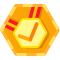## leetcode: 441. Arranging Coins 原

You have a total of n coins that you want to form in a staircase shape, where every k-th row must have exactly k coins.

Given n, find the total number of full staircase rows that can be formed.

n is a non-negative integer and fits within the range of a 32-bit signed integer.

Example 1:

```n = 5

The coins can form the following rows:
¤
¤ ¤
¤ ¤

Because the 3rd row is incomplete, we return 2.```

Example 2:

```n = 8

The coins can form the following rows:
¤
¤ ¤
¤ ¤ ¤
¤ ¤

Because the 4th row is incomplete, we return 3.```

Subscribe to see which companies asked this question.

``````# -*- coding:utf-8 -*-
# leetcode solutions
# 441. Arranging Coins
# coding by Dennis Lu
# 2017/02/11

class Solution(object):
def arrangeCoins(self, n):
"""
:type n: int
:rtype: int
"""

count = 0
row_point = 2
total = 1

while(total <= n):
total += row_point
row_point += 1
count += 1
return count``````

``````# -*- coding:utf-8 -*-
# leetcode solutions
# 441. Arranging Coins
# coding by Dennis Lu
# 2017/02/11

class Solution(object):
def arrangeCoins(self, n):
"""
:type n: int
:rtype: int
"""

first = 1
last = n
mid = int((n + 1) / 2)

while(1):
num0 = (1 + mid) * mid * 0.5
num1 = (1 + mid + 1) * (mid + 1) * 0.5

if((num0 <= n) and (num1 > n)):
return mid
if(num0 > n):
last = mid - 1
mid = int((first + last) / 2)
continue
if(num1 <= n):
first = mid + 1
mid = int((first + last) / 2)``````

``````# -*- coding:utf-8 -*-
# leetcode solutions
# 441. Arranging Coins
# coding by Dennis Lu
# 2017/02/11

import math

class Solution(object):
def arrangeCoins(self, n):
"""
:type n: int
:rtype: int
"""

return int(math.sqrt(2 * n + 0.25) - 0.5)``````

3的算法时间复杂度O(1)### 开源中国首席精神砖家Leetcode 441. Arranging Coins

SnailTyan
2018/08/31
0
0
LeetCode算法题-Arranging Coins（Java实现）

01/13
0
0

qq_32690999
2018/02/09
0
0
【DP】518. Coin Change 2

06/08
0
0

2017/06/27
21
0

SpringBoot 集成MongoDB

zw965
14分钟前
10
0

> 原文链接：使用 Envoy 和 AdGuard Home 阻挡烦人的广告 通常我们使用网络时，宽带运营商会为我们分配一个 DNS 服务器。这个 DNS 通常是最快的，距离最近的服务器，但会有很多问题，比如： ...

47分钟前
14
0
springboot之全局处理异常封装

springboot之全局处理异常封装 简介 在项目中经常出现系统异常的情况，比如NullPointerException等等。如果默认未处理的情况下，springboot会响应默认的错误提示，这样对用户体验不是友好，系...

Purgeyao
58分钟前
22
0

23
0
PHP常用函数

<?php/** * 获取客户端IP * @return [string] [description] */function getClientIp() { \$ip = NULL; if (isset(\$_SERVER['HTTP_X_FORWARDED_FOR'])) { \$arr = explode('......

14
0